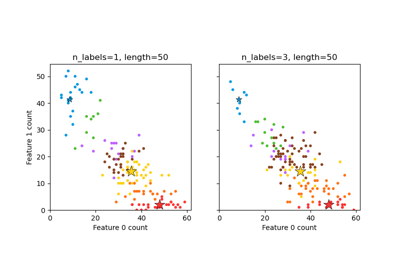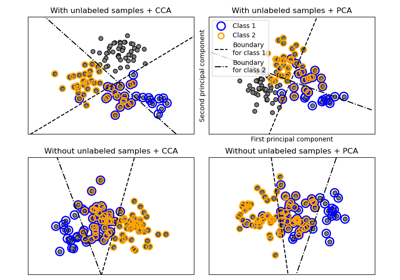# sklearn.datasets.make_multilabel_classification¶

sklearn.datasets.make_multilabel_classification(n_samples=100, n_features=20, *, n_classes=5, n_labels=2, length=50, allow_unlabeled=True, sparse=False, return_indicator='dense', return_distributions=False, random_state=None)[source]

Generate a random multilabel classification problem.

For each sample, the generative process is:
• pick the number of labels: n ~ Poisson(n_labels)

• n times, choose a class c: c ~ Multinomial(theta)

• pick the document length: k ~ Poisson(length)

• k times, choose a word: w ~ Multinomial(theta_c)

In the above process, rejection sampling is used to make sure that n is never zero or more than n_classes, and that the document length is never zero. Likewise, we reject classes which have already been chosen.

Read more in the User Guide.

Parameters
n_samplesint, default=100

The number of samples.

n_featuresint, default=20

The total number of features.

n_classesint, default=5

The number of classes of the classification problem.

n_labelsint, default=2

The average number of labels per instance. More precisely, the number of labels per sample is drawn from a Poisson distribution with n_labels as its expected value, but samples are bounded (using rejection sampling) by n_classes, and must be nonzero if allow_unlabeled is False.

lengthint, default=50

The sum of the features (number of words if documents) is drawn from a Poisson distribution with this expected value.

allow_unlabeledbool, default=True

If True, some instances might not belong to any class.

sparsebool, default=False

If True, return a sparse feature matrix

New in version 0.17: parameter to allow sparse output.

return_indicator{‘dense’, ‘sparse’} or False, default=’dense’

If 'dense' return Y in the dense binary indicator format. If 'sparse' return Y in the sparse binary indicator format. False returns a list of lists of labels.

return_distributionsbool, default=False

If True, return the prior class probability and conditional probabilities of features given classes, from which the data was drawn.

random_stateint, RandomState instance or None, default=None

Determines random number generation for dataset creation. Pass an int for reproducible output across multiple function calls. See Glossary.

Returns
Xndarray of shape (n_samples, n_features)

The generated samples.

Y{ndarray, sparse matrix} of shape (n_samples, n_classes)

The label sets. Sparse matrix should be of CSR format.

p_cndarray of shape (n_classes,)

The probability of each class being drawn. Only returned if return_distributions=True.

p_w_cndarray of shape (n_features, n_classes)

The probability of each feature being drawn given each class. Only returned if return_distributions=True.

## Examples using sklearn.datasets.make_multilabel_classification¶Plot randomly generated multilabel dataset¶Multilabel classification¶